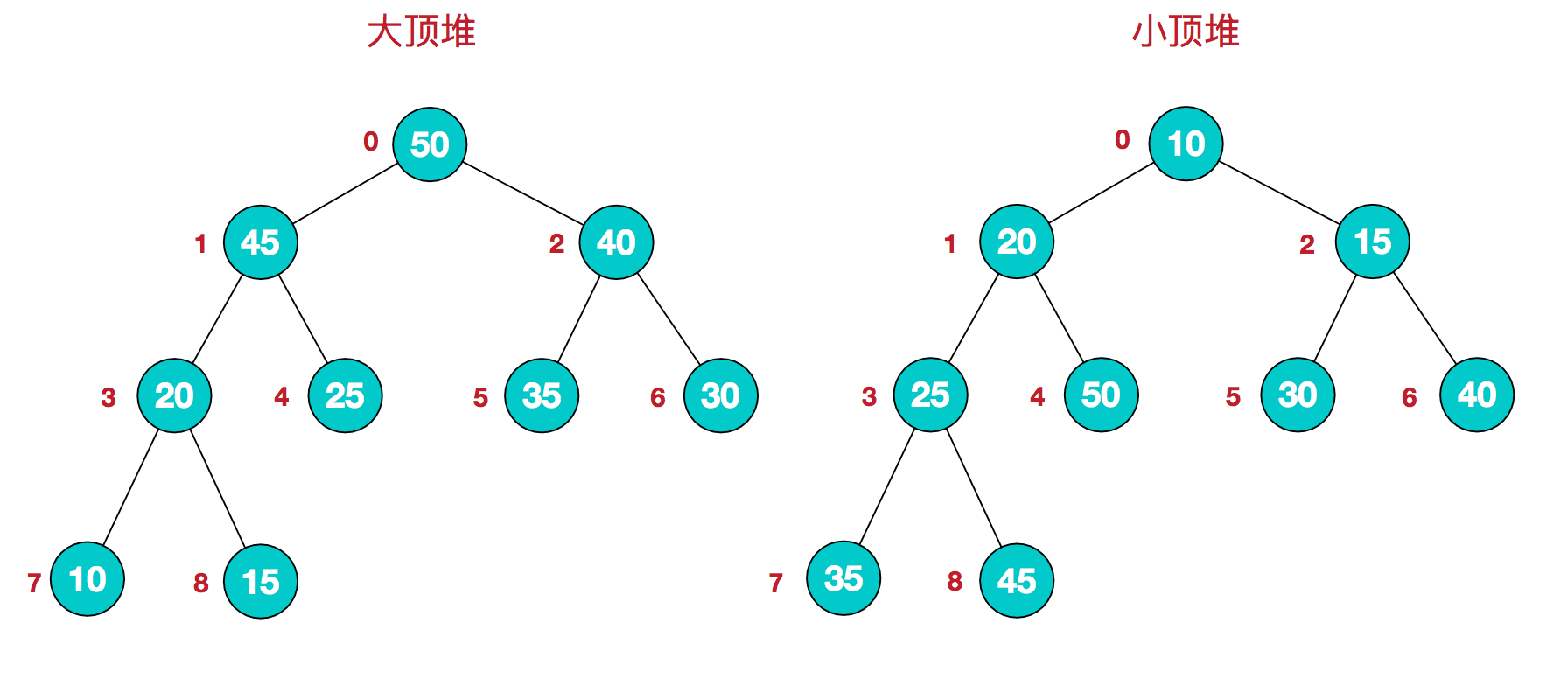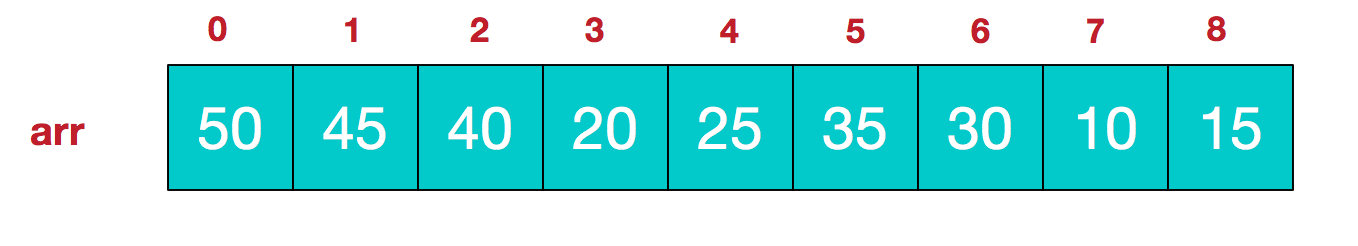# 堆#### 完全二叉树与数组的关联¶

       1
/   \
2     3
/ \   /
4   5  6


1. 索引计算方便：给定一个节点在数组中的索引 $$i$$，可以通过简单的计算得到它的父节点、左孩子和右孩子在数组中的位置。父节点的索引为 $$i/2$$，左孩子的索引为 $$2i$$，右孩子的索引为 $$2i+1$$。对于有 $$n$$ 个元素的完全二叉树（$$n>2$$），它的最后一个非叶子结点的下标：$$n/2 - 1$$
2. 节省空间：相比于使用指针来表示节点间的连接关系，数组表示使用的空间更少。

##### 代码实现（基于数组）¶
class MaxHeap {
public:
MaxHeap(int _totalSize) {
_size = 0;
_totalSize = _totalSize;
_data = new int[_totalSize + 1];
};
~MaxHeap() {
delete[] _data;
}
bool push(int element) {
_size++;
if (_size > _totalSize) {
_size--;
return false;
}
_data[_size] = element;
int index = _size;
int parent = _size / 2;
while (_data[index] > _data[parent] && index > 1) {
swap(_data[index], _data[parent]);
index = parent;
parent = index / 2;
}
return true;
}
bool pop() {
if (_size < 1) {
return false;
}
_data = _data[_size];
_size--;
int index = 1;
while (index < _size && index <= _size / 2) {
int leftChildIndex = index * 2;
int rightChildIndex = index * 2 + 1;
if (_data[index] < _data[leftChildIndex] || _data[index] <  _data[rightChildIndex]) {
if (_data[leftChildIndex] > _data[rightChildIndex]) {
swap(_data[leftChildIndex], _data[index]);
index = leftChildIndex;
} else {
swap(_data[rightChildIndex], _data[index]);
index = rightChildIndex;
}
} else {
break;
}
}
return true;
}
int size() {
return _size;
}
int top() {
if (_size == 0) return INT_MIN;
return _data;
}
private:
int _size;
int _totalSize;
int * _data;
};